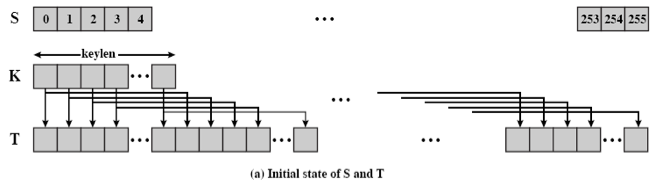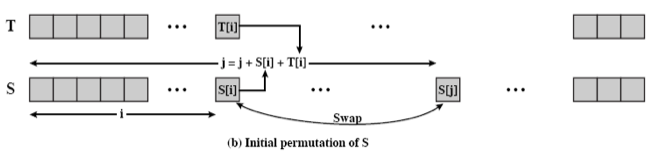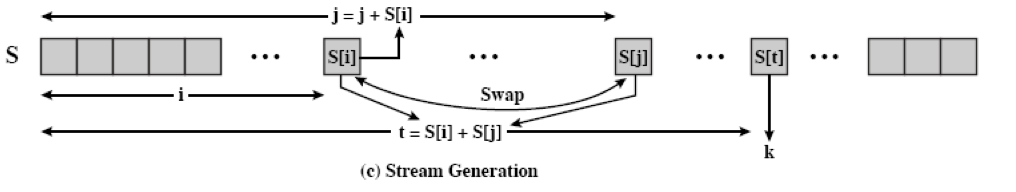# RC4¶

Warning

The current page still doesn't have a translation for this language.

Besides, you can also help to translate it: Contributing.

## 基本介绍¶

RSA 由 Ron Rivest 设计，最初隶属于 RSA 安全公司，是一个专利密码产品。它是面向字节的流密码，密钥长度可变，非常简单，但也很有效果。RC4 算法广泛应用于 SSL/TLS 协议和 WEP/WPA 协议。

## 基本流程¶

RC4 主要包含三个流程

• 初始化 S 和 T 数组。
• 初始化置换 S。
• 生成密钥流。

### 初始化 S 和 T 数组¶

for i = 0 to 255 do
S[i] = i
T[i] = K[i mod keylen])### 初始化置换 S¶

j = 0
for i = 0 to 255 do
j = (j + S[i] + T[i]) (mod 256)
swap (S[i], S[j])### 生成流密钥¶

i = j = 0
for each message byte b
i = (i + 1) (mod 256)
j = (j + S[i]) (mod 256)
swap(S[i], S[j])
t = (S[i] + S[j]) (mod 256)
print S[t]